##### Linear mixed models: a balanced lattice squareKanchana Punyawaew

8 months agoThis blog illustrates how to analyze data from a field experiment with a balanced lattice square design using linear mixed models. We’ll consider two models: the balanced lattice square model and a spatial model.

The example data are from a field experiment conducted at Slate Hall Farm, UK, in 1976 (Gilmour et al., 1995). The experiment was set up to compare the performance of 25 varieties of barley and was designed as a balanced lattice square with six replicates laid out in a 10 x 15 rectangular grid. Each replicate contained exactly one plot for every variety. The variety grown in each plot, and the coding of the replicates and lattice blocks, is shown in the field layout below: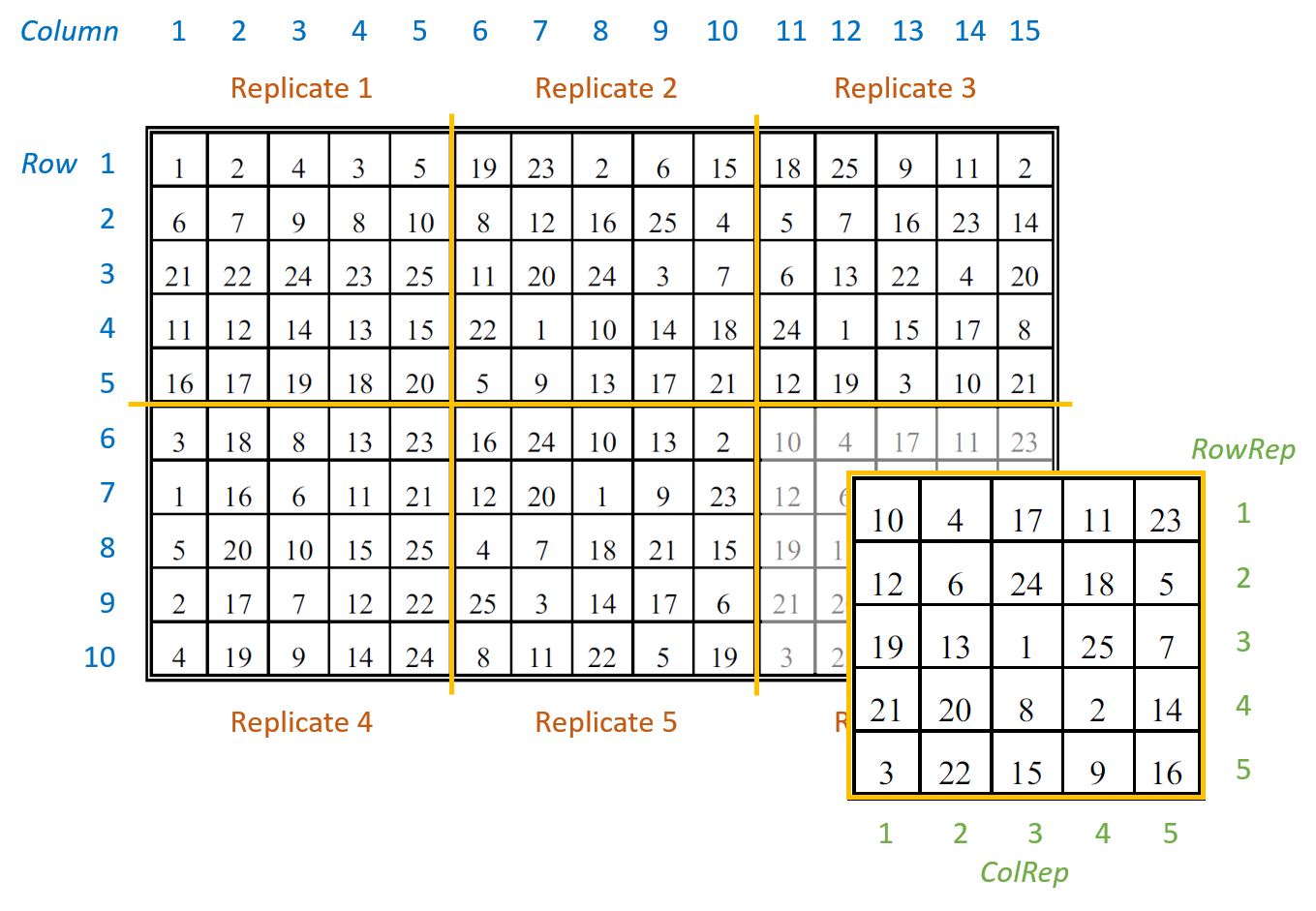There are seven columns in the data frame: five blocking factors (Rep, RowRep, ColRep, Row, Column), one treatment factor, Variety, and the response variate, yield.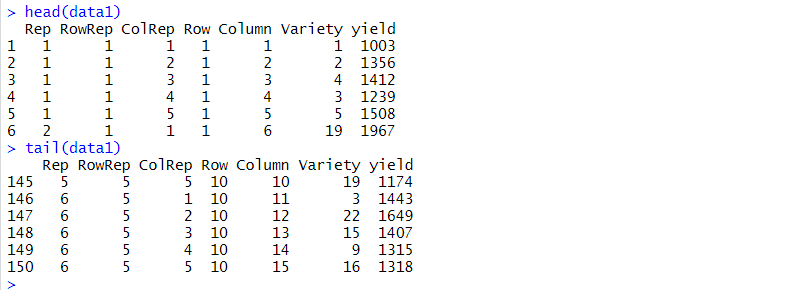The six replicates are numbered from 1 to 6 (Rep). The lattice block numbering is coded within replicates. That is, within each replicates the lattice rows (RowRep) and lattice columns (ColRep) are both numbered from 1 to 5. The Row and Column factors define the row and column positions within the field (rather than within each replicate).

### Analysis of a balanced lattice square design

To analyze the response variable, yield, we need to identify the two basic components of the experiment: the treatment structure and the blocking (or design) structure. The treatment structure consists of the set of treatments, or treatment combinations, selected to study or to compare. In our example, there is one treatment factor with 25 levels, Variety (i.e. the 25 different varieties of barley). The blocking structure of replicates (Rep), lattice rows within replicates (Rep:RowRep), and lattice columns within replicates (Rep:ColRep) reflects the balanced lattice square design. In a mixed model analysis, the treatment factors are (usually) fitted as fixed effects and the blocking factors as random.

The balanced lattice square model is fitted in ASReml-R4 using the following code:

> lattice.asr <- asreml(fixed = yield ~ Variety,
random = ~ Rep + Rep:RowRep + Rep:ColRep,
data=data1)

The REML log-likelihood is -707.786.

The model’s BIC is: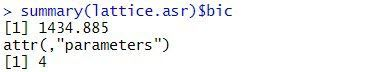The estimated variance components are: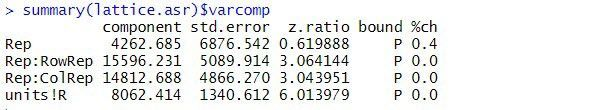The table above contains the estimated variance components for all terms in the random model. The variance component measures the inherent variability of the term, over and above the variability of the sub-units of which it is composed. The variance components for Rep, Rep:RowRep and Rep:ColRep are estimated as 4263, 15596, and 14813, respectively. As is typical, the largest unit (replicate) is more variable than its sub-units (lattice rows and columns within replicates). The "units!R" component is the residual variance.

By default, fixed effects in ASReml-R4 are tested using sequential Wald tests: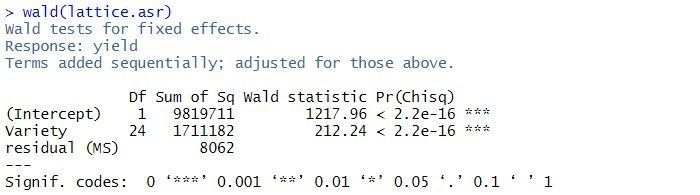In this example, there are two terms in the summary table: the overall mean, (Intercept), and Variety. As the tests are sequential, the effect of the Variety is assessed by calculating the change in sums of squares between the two models (Intercept)+Variety and (Intercept). The p-value (Pr(Chisq)) of  < 2.2 x 10-16 indicates that Variety is a highly significant.

The predicted means for the Variety can be obtained using the predict() function. The standard error of the difference between any pair of variety means is 62. Note: all variety means have the same standard error as the design is balanced.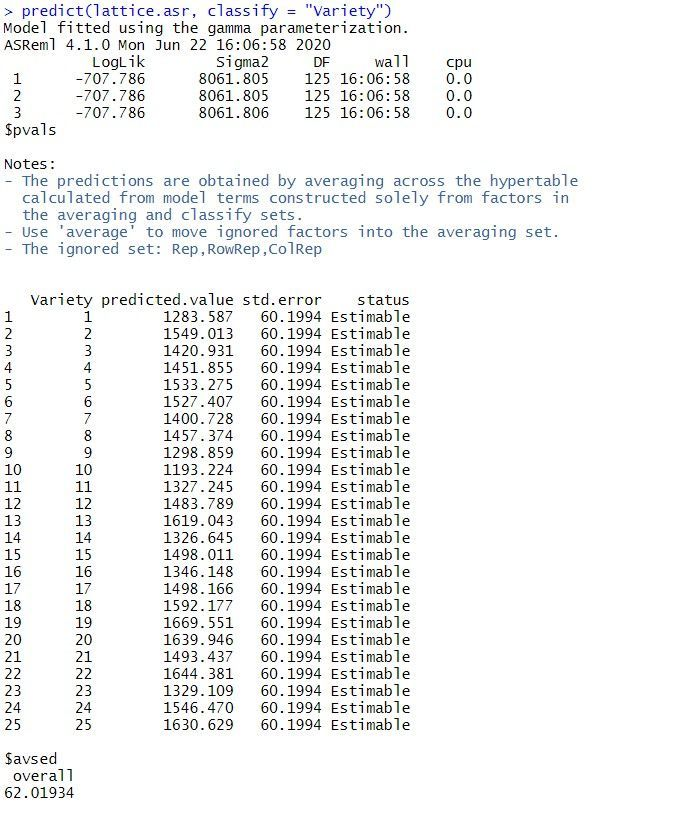Note: the same analysis is obtained when the random model is redefined as replicates (Rep), rows within replicates (Rep:Row) and columns within replicates (Rep:Column).

### Spatial analysis of a field experiment

As the plots are laid out in a grid, the data can also be analyzed using a spatial model. We’ll illustrate spatial analysis by fitting a model with a separable first order autoregressive process in the field row (Row) and field column (Column) directions. This is often a useful model to start the spatial modeling process.

The separable first order autoregressive spatial model is fitted in ASReml-R4 using the following code:

> spatial.asr <- asreml(fixed = yield ~ Variety,
residual = ~ar1(Row):ar1(Column),
data = data1)

The BIC for this spatial model is: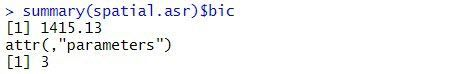The estimated variance components and sequential Wald tests are: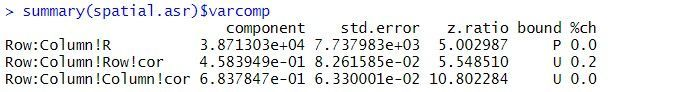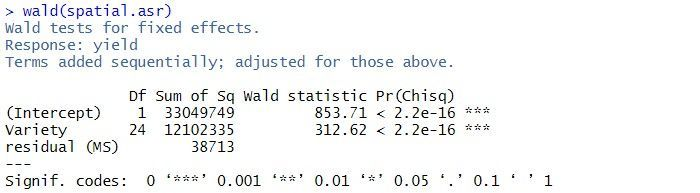The residual variance is 38713, the estimated row correlation is 0.458, and the estimated column correlation is 0.684. As for the balanced lattice square model, there is strong evidence of a Variety effect (p-value < 2.2 x 10-16).

A log-likelihood ratio test cannot be used to compare the balanced lattice square model with the spatial models, as the variance models are not nested. However, the two models can be compared using BIC. As the spatial model has a smaller BIC (1415) than the balanced lattice square model (1435), of the two models explored in this blog, it is chosen as the preferred model. However, selecting the optimal spatial model can be difficult. The current spatial model can be extended by including measurement error (or nugget effect) or revised by selecting a different variance model for the spatial effects.

#### References

Butler, D.G., Cullis, B.R., Gilmour, A. R., Gogel, B.G. and Thompson, R. (2017). ASReml-R Reference Manual Version 4. VSN International Ltd, Hemel Hempstead, HP2 4TP UK.

Gilmour, A. R., Anderson, R. D. and Rae, A. L. (1995). The analysis of binomial data by a generalised linear mixed model, Biometrika 72: 593-599..The VSNi Team

6 months ago
##### Evolution of statistical computing

It is widely acknowledged that the most fundamental developments in statistics in the past 60+ years are driven by information technology (IT). We should not underestimate the importance of pen and paper as a form of IT but it is since people start using computers to do statistical analysis that we really changed the role statistics plays in our research as well as normal life.

In this blog we will give a brief historical overview, presenting some of the main general statistics software packages developed from 1957 onwards. Statistical software developed for special purposes will be ignored. We also ignore the most widely used ‘software for statistics’ as Brian Ripley (2002) stated in his famous quote: “Let’s not kid ourselves: the most widely used piece of software for statistics is Excel.” Our focus is some of the packages developed by statisticians for statisticians, which are still evolving to incorporate the latest development of statistics.

### Ronald Fisher’s Calculating Machines

Pioneer statisticians like Ronald Fisher started out doing their statistics on pieces of paper and later upgraded to using calculating machines. Fisher bought the first Millionaire calculating machine when he was heading Rothamsted Research’s statistics department in the early 1920s. It cost about £200 at that time, which is equivalent in purchasing power to about £9,141 in 2020. This mechanical calculator could only calculate direct product, but it was very helpful for the statisticians at that time as Fisher mentioned: "Most of my statistics has been learned on the machine." The calculator was heavily used by Fisher’s successor Frank Yates (Head of Department 1933-1968) and contributed to much of Yates’ research, such as designs with confounding between treatment interactions and blocks, or split plots, or quasi-factorials.Frank Yates

Rothamsted Annual Report for 1952: "The analytical work has again involved a very considerable computing effort."

### Beginning of the Computer Age

From the early 1950s we entered the computer age. The computer at this time looked little like its modern counterpart, whether it was an Elliott 401 from the UK or an IBM 700/7000 series in the US. Although the first documented statistical package, BMDP, was developed starting in 1957 for IBM mainframes at the UCLA Health Computing Facility, on the other side of the Atlantic Ocean statisticians at Rothamsted Research began their endeavours to program on an Elliot 401 in 1954.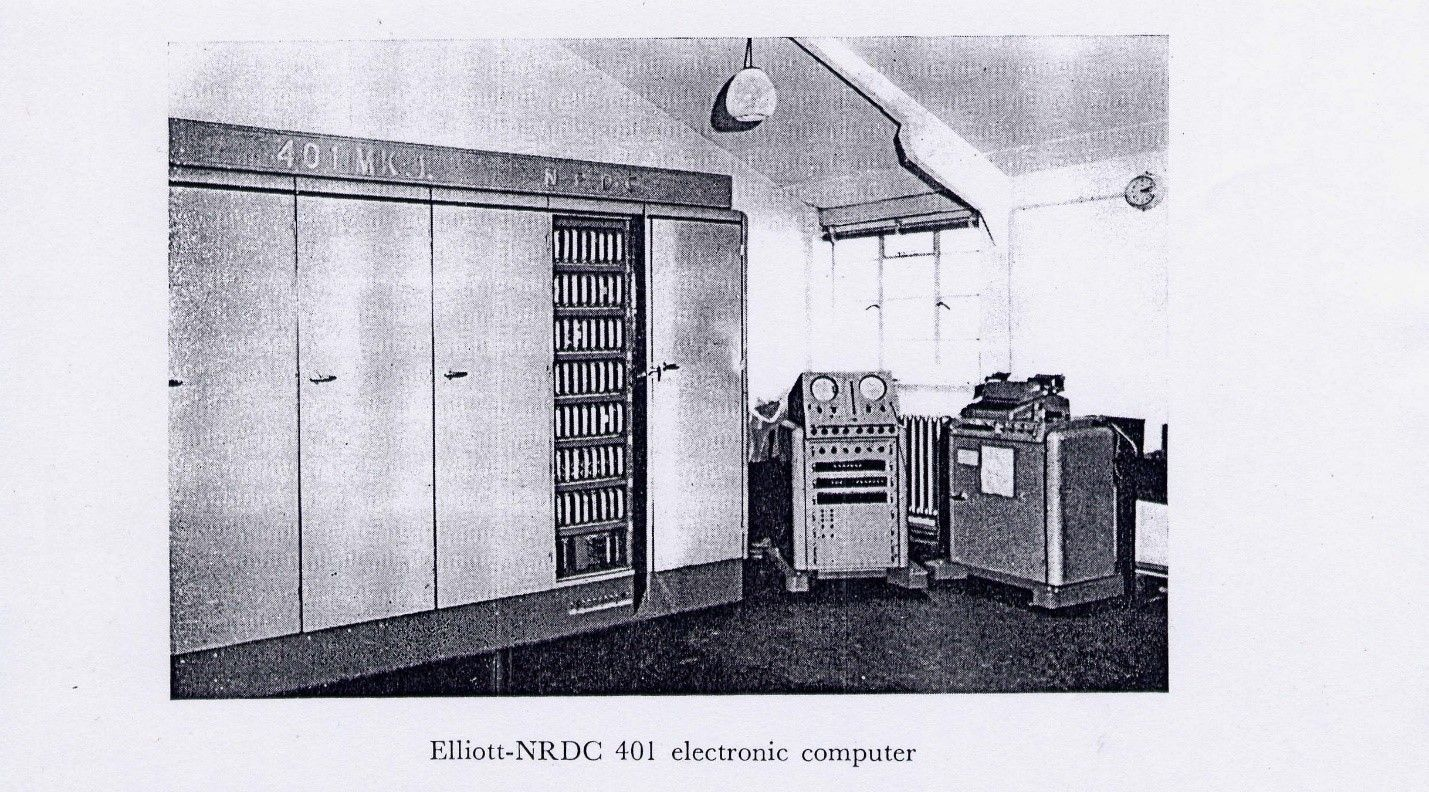### Programming Statistical Software

When we teach statistics in schools or universities, students very often complain about the difficulties of programming. Looking back at programming in the 1950s will give modern students an appreciation of how easy programming today actually is!

An Elliott 401 served one user at a time and requested all input on paper tape (forget your keyboard and intelligent IDE editor). It provided the output to an electric typewriter. All programming had to be in machine code with the instructions and data on a rotating disk with 32-bit word length, 5 "words" of fast-access store, 7 intermediate access tracks of 128 words, 16 further tracks selectable one at a time (= 2949 words – 128 for system).Computer paper tape

fitting constants to main effects and interactions in multi-way tables (1957), regression and multiple regression (1956), fitting many standard curves as well as multivariate analysis for latent roots and vectors (1955).

Although it sounds very promising with the emerging of statistical programs for research, routine statistical analyses were also performed and these still represented a big challenge, at least computationally. For example, in 1963, which was the last year with the Elliott 401 and Elliott 402 computers, Rothamsted Research statisticians analysed 14,357 data variables, and this took them 4,731 hours to complete the job. It is hard to imagine the energy consumption as well as the amount of paper tape used for programming. Probably the paper tape (all glued together) would be long enough to circle the equator.

### Development of Statistical Software: Genstat, SAS, SPSS

The above collection of programs was mainly used for agricultural research at Rothamsted and was not given an umbrella name until John Nelder became Head of the Statistics Department in 1968. The development of Genstat (General Statistics) started from that year and the programming was done in FORTRAN, initially on an IBM machine. In that same year, at North Carolina State University, SAS (Statistical Analysis Software) was almost simultaneously developed by computational statisticians, also for analysing agricultural data to improve crop yields. At around the same time, social scientists at the University of Chicago started to develop SPSS (Statistical Package for the Social Sciences). Although the three packages (Genstat, SAS and SPSS) were developed for different purposes and their functions diverged somewhat later, the basic functions covered similar statistical methodologies.

The first version of SPSS was released in 1968. In 1970, the first version of Genstat was released with the functions of ANOVA, regression, principal components and principal coordinate analysis, single-linkage cluster analysis and general calculations on vectors, matrices and tables. The first version of SAS, SAS 71, was released and named after the year of its release. The early versions of all three software packages were written in FORTRAN and designed for mainframe computers.

Since the 1980s, with the breakthrough of personal computers, a second generation of statistical software began to emerge. There was an MS-DOS version of Genstat (Genstat 4.03) released with an interactive command line interface in 1980.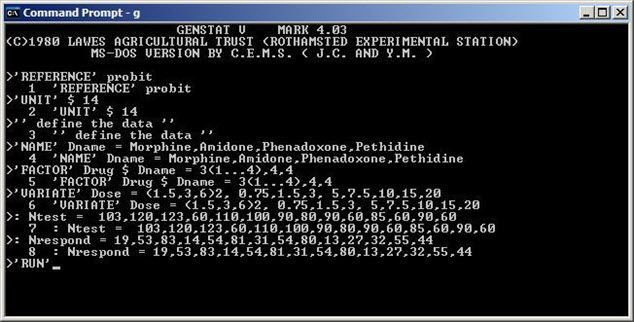Genstat 4.03 for MSDOS

Around 1985, SAS and SPSS also released a version for personal computers. In the 1980s more players entered this market: STATA was developed from 1985 and JMP was developed from 1989. JMP was, from the very beginning, for Macintosh computers. As a consequence, JMP had a strong focus on visualization as well as graphics from its inception.

### The Rise of the Statistical Language R

The development of the third generation of statistical computing systems had started before the emergence of software like Genstat 4.03e or SAS 6.01. This development was led by John Chambers and his group in Bell Laboratories since the 1970s. The outcome of their work is the S language. It had been developed into a general purpose language with implementations for classical as well as modern statistical inferences. S language was freely available, and its audience was mainly sophisticated academic users. After the acquisition of S language by the Insightful Corporation and rebranding as S-PLUS, this leading third generation statistical software package was widely used in both theoretical and practical statistics in the 1990s, especially before the release of a stable beta version of the free and open-source software R in the year 2000. R was developed by Ross Ihaka and Robert Gentleman at the University of Auckland, New Zealand, and is currently widely used by statisticians in academia and industry, together with statistical software developers, data miners and data analysts.

Software like Genstat, SAS, SPSS and many other packages had to deal with the challenge from R. Each of these long-standing software packages developed an R interface R or even R interpreters to anticipate the change of user behaviour and ever-increasing adoption of the R computing environment. For example, SAS and SPSS have some R plug-ins to talk to each other. VSNi’s ASReml-R software was developed for ASReml users who want to run mixed model analysis within the R environment, and at the present time there are more ASReml-R users than ASReml standalone users. Users who need reliable and robust mixed effects model fitting adopted ASReml-R as an alternative to other mixed model R packages due to its superior performance and simplified syntax. For Genstat users, msanova was also developed as an R package to provide traditional ANOVA users an R interface to run their analysis.

### What’s Next?

We have no clear idea about what will represent the fourth generation of statistical software. R, as an open-source software and a platform for prototyping and teaching has the potential to help this change in statistical innovation. An example is the R Shiny package, where web applications can be easily developed to provide statistical computing as online services. But all open-source and commercial software has to face the same challenges of providing fast, reliable and robust statistical analyses that allow for reproducibility of research and, most importantly, use sound and correct statistical inference and theory, something that Ronald Fisher will have expected from his computing machine!The VSNi Team

5 months ago
##### What is a p-value?

A way to decide whether to reject the null hypothesis (H0) against our alternative hypothesis (H1) is to determine the probability of obtaining a test statistic at least as extreme as the one observed under the assumption that H0 is true. This probability is referred to as the “p-value”. It plays an important role in statistics and is critical in most biological research.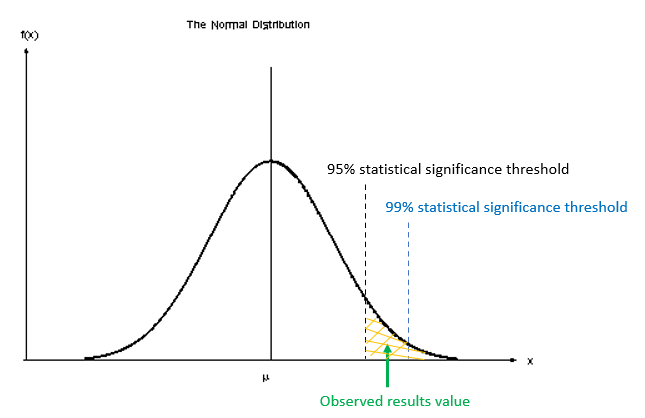#### What is the true meaning of a p-value and how should it be used?

P-values are a continuum (between 0 and 1) that provide a measure of the strength of evidence against H0. For example, a value of 0.066, will indicate that there is a probability that we could observe values as large or larger than our critical value with a probability of 6.6%. Note that this p-value is NOT the probability that our alternative hypothesis is correct, it is only a measure of how likely or unlikely we are to observe these extreme events, under repeated sampling, in reference to our calculated value. Also note that this p-value is obtained based on an assumed distribution (e.g., t-distribution for a t-test); hence, p-value will depend strongly on your (correct or incorrect) assumptions.

The smaller the p-value, the stronger the evidence for rejecting H0. However, it is difficult to determine what a small value really is. This leads to the typical guidelines of: p < 0.001 indicating very strong evidence against H0, p < 0.01 strong evidence, p < 0.05 moderate evidence, p < 0.1 weak evidence or a trend, and p ≥ 0.1 indicating insufficient evidence , and a strong debate on what this threshold should be. But declaring p-values as being either significant or non-significant based on an arbitrary cut-off (e.g. 0.05 or 5%) should be avoided. As Ronald Fisher said:

“No scientific worker has a fixed level of significance at which, from year to year, and in all circumstances he rejects hypotheses; he rather gives his mind to each particular case in the light of his evidence and his ideas” .

A very important aspect of the p-value is that it does not provide any evidence in support of H0 – it only quantifies evidence against H0. That is, a large p-value does not mean we can accept H0. Take care not to fall into the trap of accepting H0! Similarly, a small p-value tells you that rejecting H0 is plausible, and not that H1 is correct!

For useful conclusions to be drawn from a statistical analysis, p-values should be considered alongside the size of the effect. Confidence intervals are commonly used to describe the size of the effect and the precision of its estimate. Crucially, statistical significance does not necessarily imply practical (or biological) significance. Small p-values can come from a large sample and a small effect, or a small sample and a large effect.

It is also important to understand that the size of a p-value depends critically on the sample size (as this affects the shape of our distribution). Here, with a very very large sample size, H0 may be always rejected even with extremely small differences, even if H0 is nearly (i.e., approximately) true. Conversely, with very small sample size, it may be nearly impossible to reject H0 even if we observed extremely large differences. Hence, p-values need to also be interpreted in relation to the size of the study.

#### References

 Ganesh H. and V. Cave. 2018. P-values, P-values everywhere! New Zealand Veterinary Journal. 66(2): 55-56.

 Fisher RA. 1956. Statistical Methods and Scientific Inferences. Oliver and Boyd, Edinburgh, UK.The VSNi Team

5 months ago
##### Should I drop the outliers from my analysis?

Outliers are sample observations that are either much larger or much smaller than the other observations in a dataset. Outliers can skew your dataset, so how should you deal with them?

#### An example outlier problem

Imagine Jane, the general manager of a chain of computer stores, has asked a statistician, Vanessa, to assist her with the analysis of data on the daily sales at the stores she manages. Vanessa takes a look at the data, and produces a boxplot for each of the stores as shown below.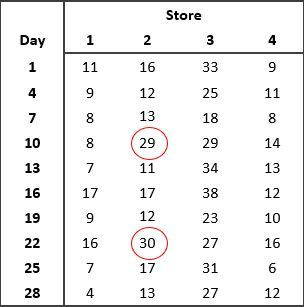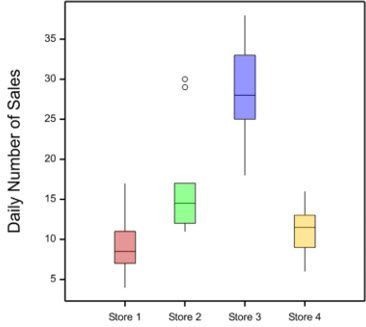#### What do you notice about the data?

Vanessa pointed out to Jane the presence of outliers in the data from Store 2 on days 10 and 22. Vanessa recommended that Jane checks the accuracy of the data. Are the outliers due to recording or measurement error? If the outliers can’t be attributed to errors in the data, Jane should investigate what might have caused the increased sales on these two particular days. Always investigate outliers - this will help you better understand the data, how it was generated and how to analyse it.

#### Should we remove the outliers?

Vanessa explained to Jane that we should never drop a data value just because it is an outlier. The nature of the outlier should be investigated before deciding what to do.

Whenever there are outliers in the data, we should look for possible causes of error in the data. If you find an error but cannot recover the correct data value, then you should replace the incorrect data value with a missing value.However, outliers can also be real observations, and sometimes these are the most interesting ones! If your outlier can’t be attributed to an error, you shouldn’t remove it from the dataset. Removing data values unnecessarily, just because they are outliers, introduces bias and may lead you to draw the wrong conclusions from your study.

#### What should we do if we need/want to keep the outlier?

• Transform the data: if the dataset is not normally distributed, we can try transforming the data to normalize it. For example, if the data set has some high-value outliers (i.e. is right skewed), the log transformation will “pull” the high values in. This often works well for count data.
• Try a different model/analysis: different analyses may make different distributional assumptions, and you should pick one that is appropriate for your data. For example, count data are generally assumed to follow a Poisson distribution. Alternatively, the outliers may be able to be modelled using an appropriate explanatory variable. For example, computer sales may increase as we approach the start of a new school year.

In our example, Vanessa suggested that since the mean for Store 2 is highly influenced by the outliers, the median, another measure of central tendency, seems more appropriate for summarizing the daily sales at each store. Using the statistical software Genstat, Vanessa can easily calculate both the mean and median number of sales per store for Jane.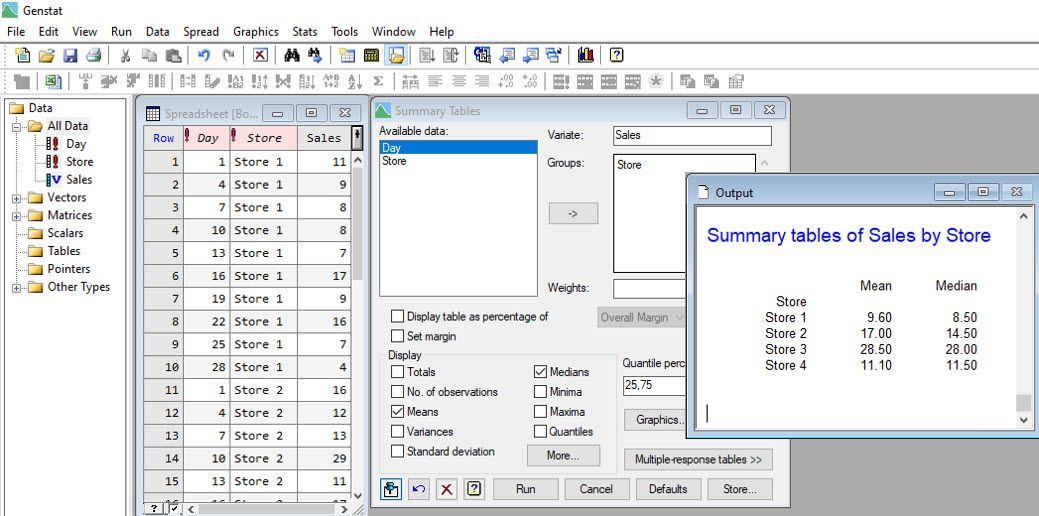Vanessa also analyses the data assuming the daily sales have Poisson distributions, by fitting a log-linear model.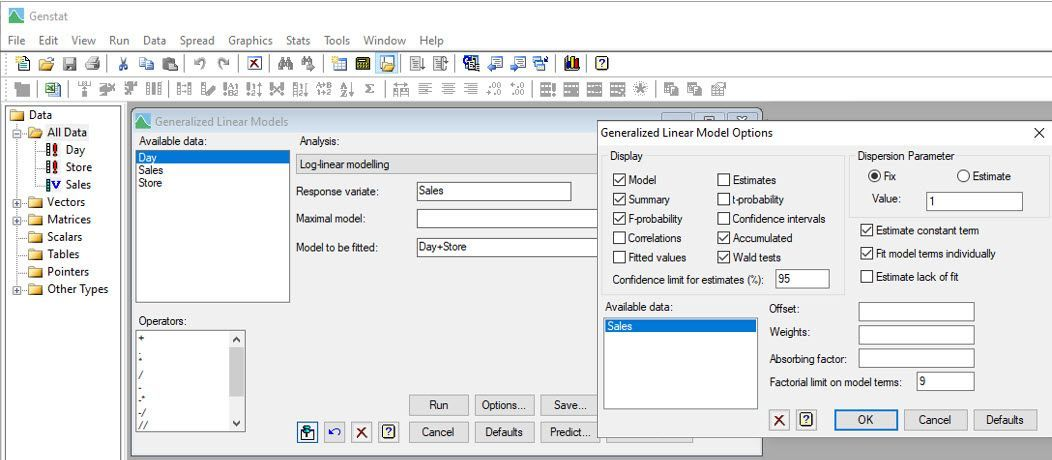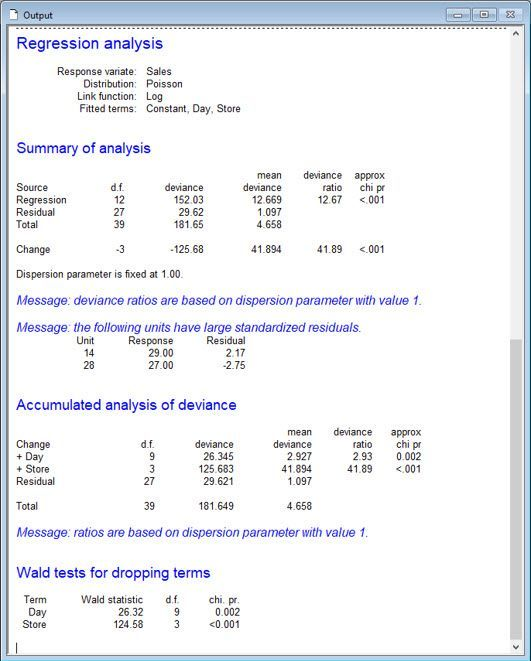Notice that Vanessa has included “Day” as a blocking factor in the model to allow for variability due to temporal effects.

From this analysis, Vanessa and Jane conclude that the means (of the Poisson distributions) differ between the stores (p-value < 0.001). Store 3, on average, has the most computer sales per day, whereas Stores 1 and 4, on average, have the least.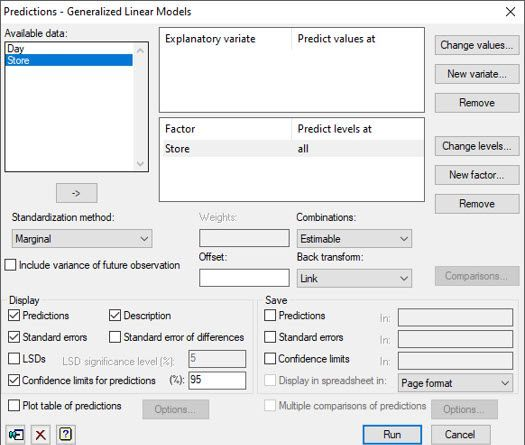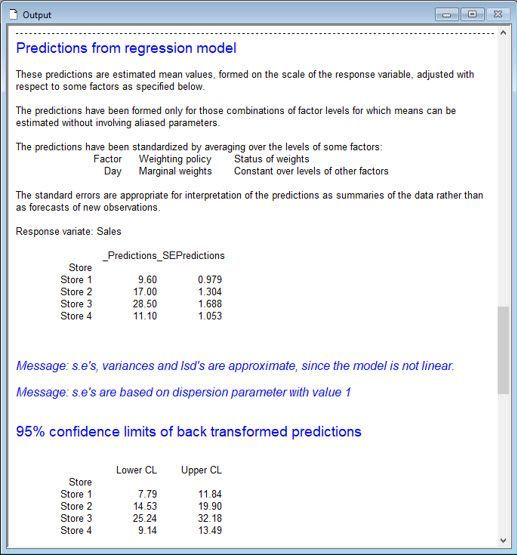There are other statistical approaches Vanessa might have used to analyse Jane’s sales data, including a one-way ANOVA blocked by Day on the log-transformed sales data and Friedman’s non-parametric ANOVA. Both approaches are available in Genstat’s comprehensive menu system.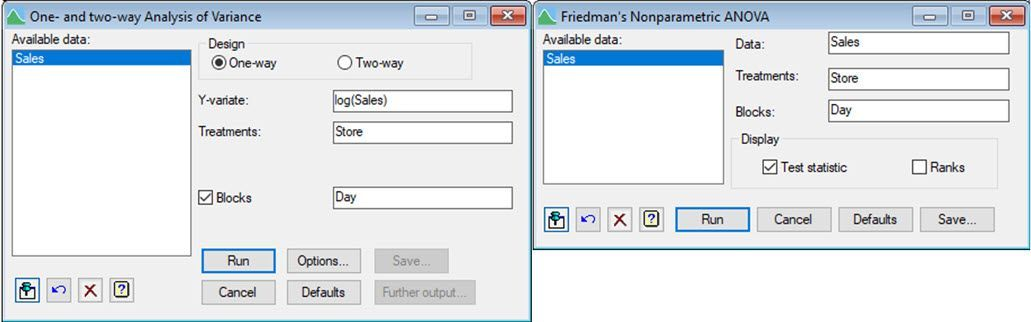#### What is the best method to deal with outliers?

There are many ways to deal with outliers, but no single method will work in every situation. As we have learnt, we can remove an observation if we have evidence it is an error. But, if that is not the case, we can always use alternative summary statistics, or even different statistical approaches, that accommodate them.